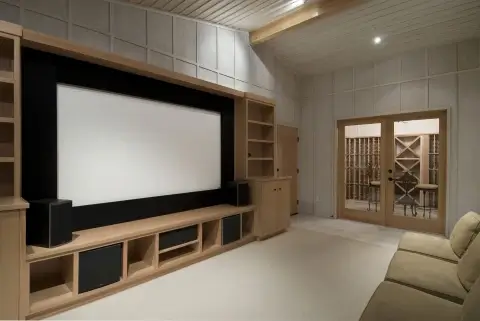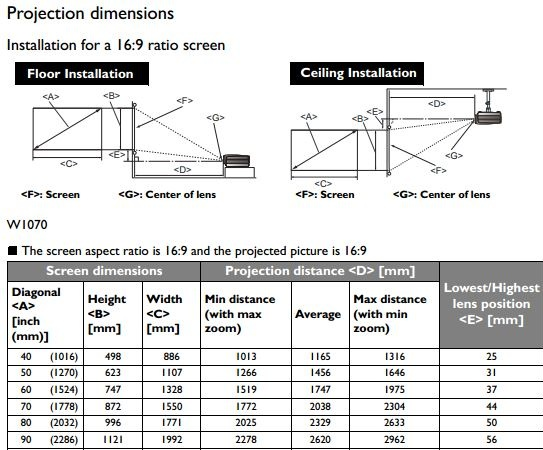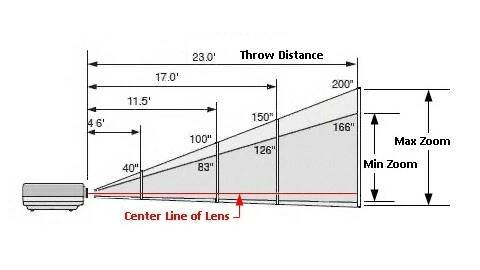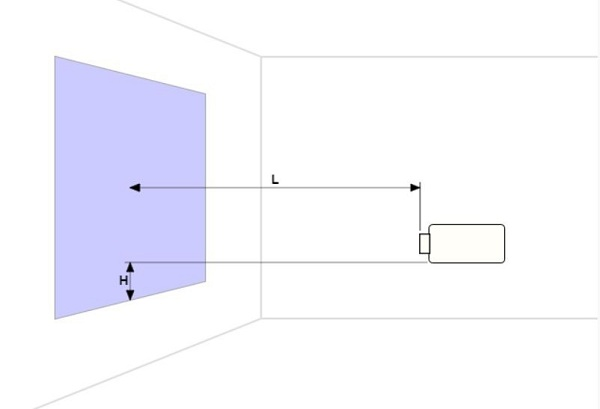# How to Calculate Projector Screen Size

## Understanding Projector Screen Size Calculations

It’s widely accepted that a projector offers a larger screen for your home theater compared to the average television. However, understanding the exact dimensions of the projector screen and how they compare can be confusing. The product description metrics are only as useful as your ability to comprehend them. This article will provide a comprehensive guide on how to calculate your projector screen size, from understanding maximum potential dimensions to the effect of changing the projector’s distance from the screen.

### Projector Screen Measurements: More Than Just Screen Width and HeightProjector screen measurements, unlike most products, are typically listed in terms of a single dimension. For TVs and projectors, the image and screen sizes are measured diagonally. If you need to figure out the projector screen size to match your projector to the right screen, or for comparison purposes, this information is usually sufficient. However, in some situations, knowing just the diagonal measurement may not be enough. >>> Try our screen size calculator.

### Unraveling the Mystery of Screen Width and HeightSource:benq.us

When you need to determine the best spot for the screen in your home, for instance, you’ll need to calculate the screen’s dimensions from the screen size. Here, your high school geometry knowledge comes into play. A diagonal drawn across a rectangular screen divides it into two right triangles, with the diagonal acting as the hypotenuse for each. However, the diagonal could represent the hypotenuse of countless right triangles. Consequently, to figure out the screen’s dimensions, you’ll need to know both the length and slope of the hypotenuse.

### Influence of Aspect Ratio on Screen Width and Height

The aspect ratio of the projector is vital at this point. It defines the relationship between the image width and height in a numerical ratio. For instance, an aspect ratio of 1:1 signifies equal width and height. If the aspect ratio is 2:1, the width is twice the height. Different content types have varying aspect ratios. HD movies and TV shows typically have a 16:9 aspect ratio, while a cinematic experience requires a 2.35:1 ratio. You can use the aspect ratio in conjunction with the Pythagorean theorem to determine the exact dimensions of the image or screen. You can also find an aspect ratio calculator online if you’re not mathematically inclined.

### The Role of Projector Throw Distance in Determining Screen SizeSource:projectorcentral.com

Unlike televisions, the screen size produced by a projector isn’t fixed. The image size created by the projector lamp varies depending on the distance between the lamp and the screen, commonly referred to as the projector throw distance. Thankfully, the aspect ratio remains constant, only the scale changes. That is, the length and width increase or decrease proportionately. The throw ratio, calculated by dividing the width of the image by the distance, can help predict how much the screen size will change when it’s moved.

### Considerations Beyond Projector Throw Distance: Zoom and Alignment

Zooming complicates the throw ratio calculation, but in general, the larger the distance between the lens and the screen, the larger the required screen. It’s also crucial to remember that alignment affects the picture as well as distance. Shifting the projector up and down or sideways in relation to the screen or altering its projection angle can impact the image’s size and quality.Source:epson.com

### Decoding Screen Gain and its Impact on Viewing Distance

Screen gain is another essential factor that determines your viewing distance. It refers to the measure of a screen’s reflectivity. A high-gain screen reflects more light towards the audience and thus appears brighter. Conversely, low-gain screens distribute light more evenly across a wider angle. The chosen gain impacts your seating arrangement and the viewing distance, ensuring optimal visual comfort.

### The Math Behind Projector Screen Size Calculation

Calculating a projector screen’s size involves a few important equations:

1. The throw ratio (T) equals the width (W) divided by the distance (D), expressed as T=W/D.
2. The aspect ratio varies depending on the content type—16:9 for HD content and 2.35:1 for cinema screens.
3. The Pythagorean Theorem, usually written as A2+B2=C2, can be thought of as W2+H2=S2 for our purposes, where W2+H2 equals the screen size2.

If you have identified a place for your projector, measure the distance from the lens to the wall and multiply this by the throw ratio. This calculation gives you the projected image’s width. Apply the aspect ratio to find the height, and then use the Pythagorean theorem to find the screen size.

Try our screen size calculator below:

## Projector Screen Size Calculator

### Using Projector Screen Size to Determine Ideal Placement

Conversely, if you have a specific area on your wall in mind for your image, you can measure this space and decide the image size. Use painter’s tape to mark out your envisioned image (using the aspect ratio to get the dimensions), then use the Pythagorean theorem to get the screen size. From this, you can divide the width by the throw ratio to calculate how far your projector needs to be.

While calculating your projector’s screen size might seem daunting initially, it becomes easier with a bit of practice. Taking the time to make these calculations for your specific space before buying and installing your equipment can save you a lot of guesswork and trouble in the long run.